# Round cake

What is the area of a round cake with a radius of 5cm?

S =  78.5 cm2

### Step-by-step explanation:Did you find an error or inaccuracy? Feel free to write us. Thank you!#### You need to know the following knowledge to solve this word math problem:

We encourage you to watch this tutorial video on this math problem:

## Related math problems and questions:

• AnnulusThe radius of the larger circle is 8cm, the radius of smaller is 5cm. Calculate the contents of the annulus.Find the radius of the circle with area S = 200 cm².Calculate the radius of the quadrant, which area is equal to area of circle with radius r = 15 cm.
• Circle - easy 2The circle has a radius 6 cm. Calculate:
• Two circlesTwo circles with a radius 4 cm and 3 cm have a center distance 0.5cm. How many common points have these circles?
• Dort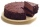The cube-shaped cube box has dimensions of 30 cm × 30 cm × 12 cm. How many CZK (Czech crowns) would cost be the biggest cake that would fit into the box when a 10 cm³ cake costs CZK 0.5? The cake has the same shape as the box.
• Right triangleA circle with a radius of 5 cm is described in a right triangle with a 6 cm leg. What is the height at the hypotenuse of this triangle?
• Round table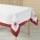A tablecloth should be sewn on a round table with a diameter of 78 cm, which should extend around the table by 10 cm. How many cms of ribbons need to be bought for edging?
• Curved surface area CSA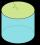A cylinder 5cm high has a base radius(7/2) cm. Calculate the curved surface area.
• Semicircle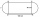Calculate the length of a semicircle with a radius of 6cm.
• Strip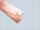From 5.9 cm wide strip should be cut rhombus with area 28 cm2. How long will be its side?
• Cake 71/3 of a cake shared with 4 people. What share of the whole cake has each people?
• Annulus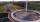Calculate the area of two circles annulus k1 (S, 3 cm) and k2 (S, 5 cm).
• A butterA butter cube with an edge 6.5 cm long is packed in a package with dimensions a = 28 cm, b = 15 cm. Calculate how many cm2 the package is larger than the surface of the cube.
• Rhombus sidesfind the sides of a rhombus, it area is 550 cm square and altitude is 55cmCalculate the radius of the circle whose length is 107 cm larger than its diameter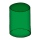I need to calculate the height of the cylinder. I have a given that the radius is 6 cm and the volume is 282.6 cm3. What is the formula for this?